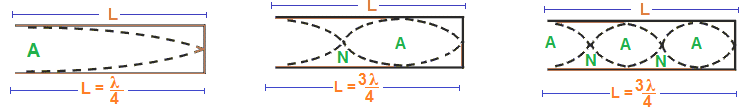# Stationary Wave in Closed Organ Pipe

The organ pipe whose one end is closed is called closed organ pipe. Whistle is an examples of closed organ pipe.

The various modes of vibration in a closed organ pipe are discussed below:Different mode of vibrations in closed pipe

Fundamental mode of vibration:

If the air inside the open organ tube oscillates and give a suitable note at resonant frequency, then this mode of vibration is called fundamental mode of vibration.  In this mode of vibration, it has one node and one antinode in its  open end as shown in first figure above.

In this mode, a node and an antinode is formed at the closed end and open end respectively due to the resonance of air column inside the pipe.

Let L is the length of the distance between a node and an antinode. It is λ/4 where λ is the wavelength of the stationary wave. Then,

$$L = \frac{\lambda }{4}$$

$$\text{or,} \lambda = 4L$$

If v is the velocity of the sound and f1 is the frequency of its vibration, then we can write:

$$v = \lambda f_1$$

$$\text{or,} f_1 = \frac{v}{\lambda } = \frac{v}{4L}$$

$$\therefore f_1 = \frac{v}{4L}$$

This is the lowest frequency produced in an open organ pipe. It is called fundamental frequency or first harmonics.

Second mode (First overtone):

If a standing sound wave pattern having two nodes and two antinodes are formed in open pipe, then such type of vibration is called second mode of vibration. In this mode, length of the pipe is equal to the wavelength of the sound as shown in second figure above i.e., λ1 = L.

If λ is the wavelength of the wave, then we have:

$$L = \frac{3\lambda }{4}$$

$$\text{or,} \lambda = \frac{4L}{3}$$

If v is the velocity of the sound and f2 is the frequency of its vibration, then we can write:

$$v = \lambda f_2$$

$$\text{or,} f_2 = \frac{v}{\lambda} = \frac{v}{\frac{4L}{3}} = \frac{3v}{4L} = 3f_1$$

$$\therefore f_2 = 3f_1$$

This is the frequency of first overtone or third harmonics. The frequency of the first overtone is three times the fundamental frequency.

Third mode (Second overtone):

There are three nodes and three antinodes in this type of vibration as shown in third figure above.

If λ is the wavelength of the wave, then we have:

$$L = \frac{5\lambda }{4}$$

$$\text{or,} \lambda = \frac{4L}{5}$$

If v is the velocity of the sound and f3 is the frequency of its vibration, then we can write:

$$v = \lambda f_3$$

$$\text{or,} f_3 = \frac{v}{\lambda} = \frac{v}{\frac{4L}{5}} = 5 \frac{v}{4L} = 5f_1$$

$$\therefore f_3 = 5f_1$$

This is the frequency of second overtone or third harmonics. The frequency of the first overtone is five times the fundamental frequency.

Conclusion:  From the above expressions, we can say that:

• The frequencies of various modes of vibration are odd integral multiple of fundamental vibration.
• The frequency of first overtone is three times the fundamental frequency.
• The frequency of second overtone is five times the fundamental frequency.
• Even harmonics are not present.
• The sound produced in this pipe has poorer quality as only odd harmonics are present in the pipe.

Do you like this article ? If yes then like otherwise dislike : 0